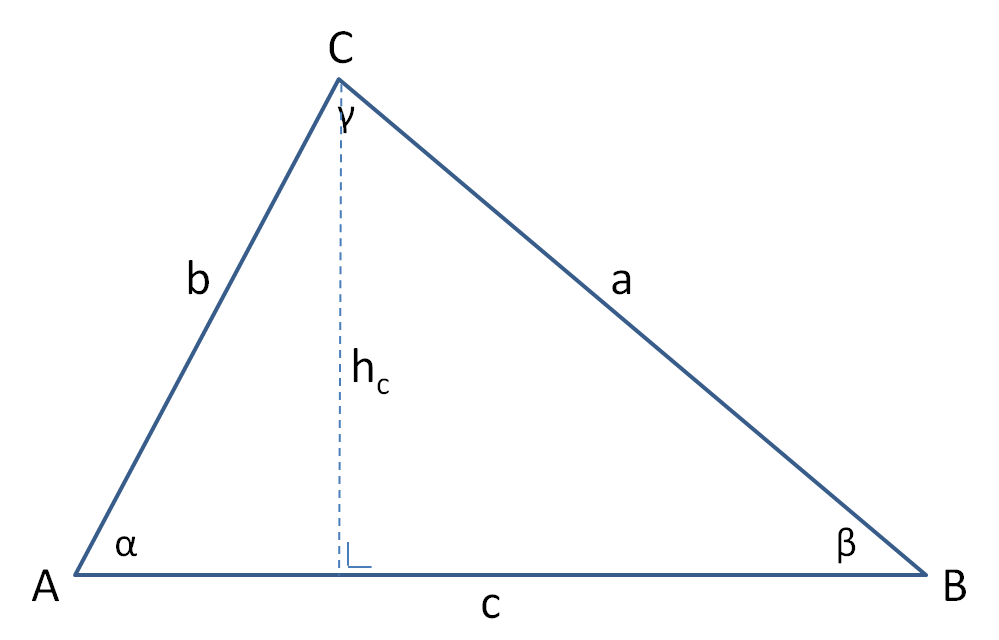# Triangle calculator

Calculate sides, angles, height and the area of a triangle with the triangle calculator.

Enter 3 values, of which at least one side. The triangle values will be calculated based on 3 input values. When more values are entered, they will be overwritten. A graphic representation of the triangle will be presented after the calculation has been made.

#### Triangle calculator

 Side a: Side b: Side c (base): Angle A (α): Angle B (β): Angle C (γ): Height (hc): Area: Perimeter : Enter 3 values, of which at least one side.

#### Triangle ABC (example)The triangle calculator uses different formulas depending on which values are known.